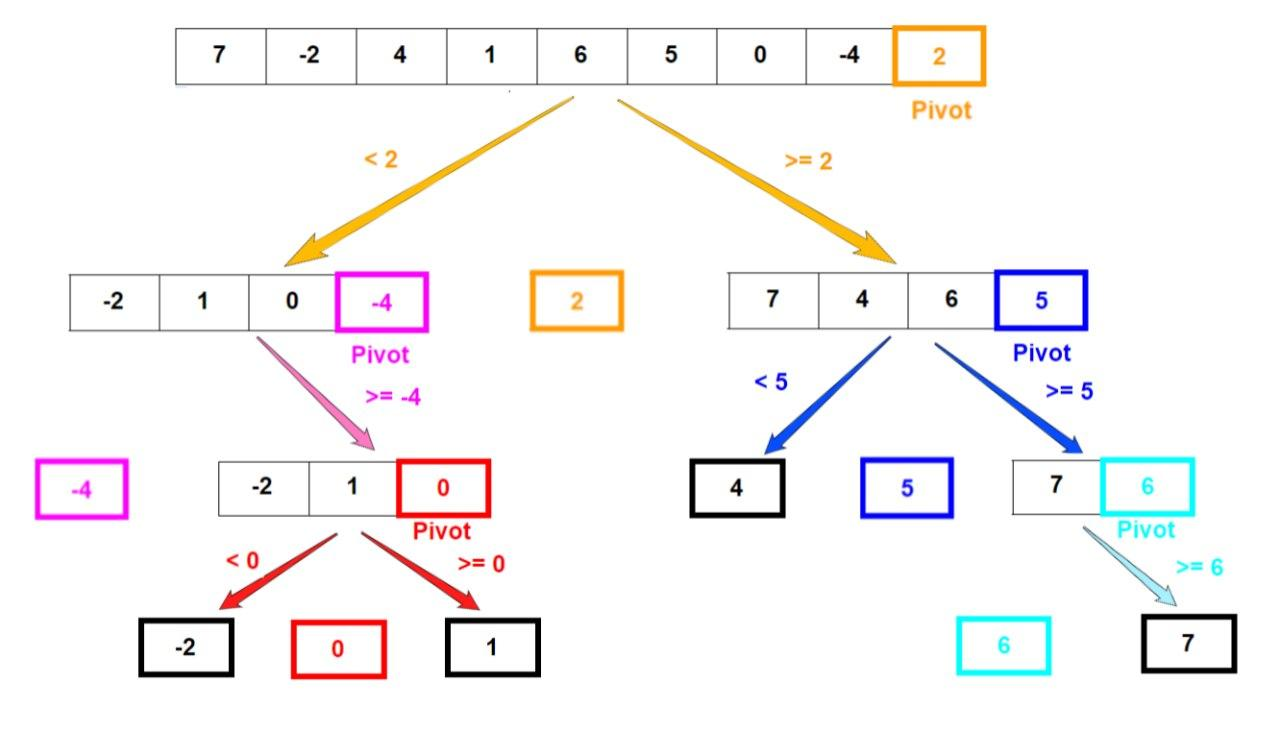# Quicksort in JavaScriptOver the years, many sorting algorithms have been developed, and one of the fastest ones to date is Quicksort. Quicksort uses the divide-and-conquer strategy to sort the given list of elements. This means that the algorithm breaks down the problem into subproblems until they become simple enough to solve directly.

#### Introduction

Sorting refers to arranging items of a list in a specific order (numerical or alphabetic). Sorting is generally used in tandem with searching.

Over the years, many sorting algorithms have been developed, and one of the fastest ones to date is Quicksort.

Quicksort uses the divide-and-conquer strategy to sort the given list of elements. This means that the algorithm breaks down the problem into subproblems until they become simple enough to solve directly.

Algorithmically this can be implemented either recursively or iteratively. However, the recursive approach is more natural for this problem.

#### Understanding the Logic Behind Quicksort

Let's take a look at how Quicksort works:

1. Select an element of the array. This element is generally called the pivot. Most often this element is either the first or the last element in the array.
2. Then, rearrange the elements of the array so that all the elements to the left of the pivot are smaller than the pivot and all the elements to the right are greater than the pivot. The step is called partitioning. If an element is equal to the pivot, it doesn't matter on which side it goes.
3. Repeat this process individually for the left and right side of the pivot, until the array is sorted.

Let's understand these steps further by walking through an example. Consider an array of unsorted elements `[7, -2, 4, 1, 6, 5, 0, -4, 2]`. We'll choose the last element to be the pivot. The step-by-step breakdown of the array would be as shown in the image below:Elements that have been chosen as a pivot in one step of the algorithm have a colored outline. After partitioning, pivot elements are always in their correct positions in the array.

Arrays that have a bolded black outline represent the end of that specific recursive branch since we ended up with an array that contained only one element.

We can see the resulting sorting of this algorithm by simply going through the lowest element in each "column".

## The essential JavaScript concepts that you should understand

The essential JavaScript concepts that you should understand - For successful developing and to pass a work interview

## Data Types In JavaScript

JavaScript data types are kept easy. While JavaScript data types are mostly similar to other programming languages; some of its data types can be unique. Here, we’ll outline the data types of JavaScript.

## JavaScript Memory Management System

The main goal of this article is help to readers to understand that how memory management system performs in JavaScript. I will use a shorthand such as GC which means Garbage Collection. When the browsers use Javascript, they need any memory location to store objects, functions, and all other things. Let’s deep in dive that how things going to work in GC.

## Create a Line Through Effect with JavaScript

In this post we are going to create an amazing line through effect, with help of CSS and lots of JavaScript. So, head over to your terminal and create a folder LineThroughEffect. Create three files -index.html, main.js and styles.css inside it.

## Grokking Call(), Apply() and Bind() Methods in JavaScript

In this article, we will have a look at the call(), apply() and bind() methods of JavaScript. Basically these 3 methods are used to control the invocation of the function.